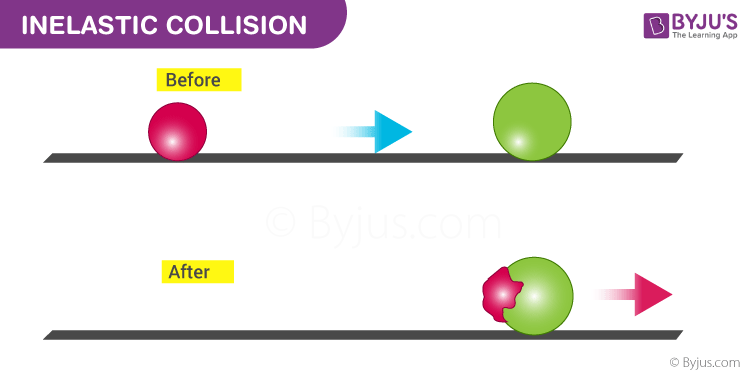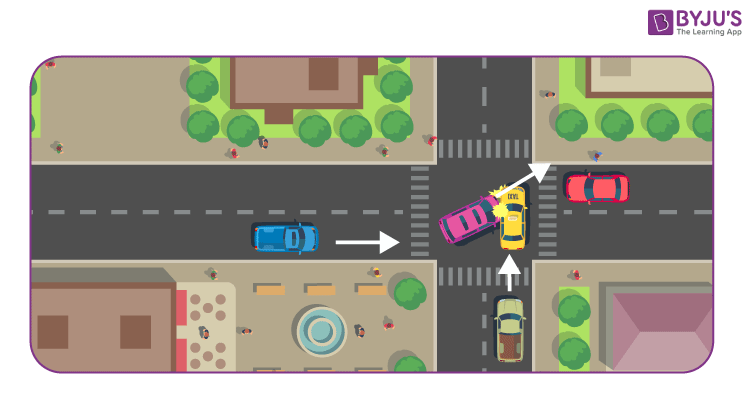# Inelastic Collision

In physics, an inelastic collision occurs when some amount of kinetic energy of a colliding object/system is lost. The colliding particles stick together, and the maximum amount of kinetic energy is lost in a perfectly inelastic collision. In such cases, kinetic energy lost is used in bonding the two bodies together. Problems involving collisions are usually solved using the conservation of momentum and energy.

## Inelastic Collision Definition

An inelastic collision is such a type of collision that takes place between two objects in which some energy is lost. In the case of inelastic collision, momentum is conserved but the kinetic energy is not conserved. Most of the collisions in daily life are inelastic in nature.The above schematic diagram illustrates a perfectly inelastic collision.
What is a collision? A collision is an event in which two or more objects exert forces on each other for a short interval of time. It is categorised into two types:

• Inelastic collision
• Elastic collision

### Perfectly Inelastic Collision

The special case of inelastic collision is known as a perfectly inelastic collision. Here, two objects stick together after collision and move as a single object. Refer to the figure above. For example, when a wet mudball is thrown against a wall, the mudball sticks to the wall.

## Inelastic Collision Formula

When two objects collide under inelastic conditions, the final velocity with which the object moves is given by-

$$\begin{array}{l}V=\frac{(M_{1}V_{1}+M_{2}V_{2})}{(M_{1}+M_{2})}\end{array}$$

Where,

• V= Final velocity
• M1= mass of the first object in kgs
• M2= mas of the second object in kgs
• V1= initial velocity of the first object in m/s
• V2= initial velocity of the second object in m/s

### Inelastic Collision in Two Dimension

For inelastic collision in two dimensions, conservation of momentum is applied separately along each axis. Since Momentum is a vector equation, there is one conservation of momentum equation per dimension. There is only one conservation of energy equation.## Inelastic Collision Examples

Most of the collision we see in our day to day life falls under inelastic collision. Some of them are listed below.

Real World Examples Of Inelastic Collision

• The ball is dropped from a certain height and it is unable to rise to its original height.
• When a soft mudball is thrown against the wall, it will stick to the wall.
• The accident of two vehicles
• A car hitting a tree

## Inelastic Collision Kinetic Energy

In the case of inelastic collision, the kinetic energy is not conserved. The loss of kinetic energy is due to internal friction. It may turn into vibrational energy of the atoms, causing a heating effect and the bodies are deformed.

Elastic Collision

Any collision in which the collided objects get separated after the collision is known as an elastic collision. In the case of elastic collision, kinetic energy gets conserved. One must use both conservations of momentum and conservation of energy to find the motions of the objects later.

Some examples of elastic collisions are ping-pong balls, billiards, etc.## Inelastic Collision Problems

#### Problem 1:

Shankha is going by a slippery snowy hill. He has a mass of 20kg, and he is sliding the hill at a velocity of 5m/s. Shankha’s elder brother has a mass of 30kg. His brother is moving slower with a velocity of 2m/s. Shankha collides to his brother. Then both of them keep going down the hill as one unit. Calculate the resulting velocity of Shankha.

#### Solution:

Given,

M1= 20kg

M2= 30kg

V1= 5m/s

V2= 2m/s

$$\begin{array}{l}V=\frac{(20kg)\times (5m/s)+(30kg)\times (2m/s)}{(20kg+30kg)}\\ \\ =\frac{100\: kg.m/s+60\: kg.m/s}{50\: kg}\\ \\ =\frac{160\: kg.m/s}{50\: kg}\\ \\ =3.20\: m/s\end{array}$$

Thus, after Shankha collides, the combined velocity of Shankha and his brother is 3.20m/s.

#### Problem 2:

Shankha driving a 20000 kg truck and travelling eastwards at a speed of 10m/s. He hit the rear end of a 1400kg car at the stop signal. The collision caused both vehicles to stick together. What is the final momentum of the vehicle?

#### Solution:

Given,

Mt = 20000kg

Mc= -1400kg

Vt =10m/s

Vc = 0m/s

Formula : Momentum P = MV

According to the conservation of momentum Pi = Pf

(MV)t+(MV)c = (Mt+Mc)V

(20000×10)(1400×0)=(20000+1400)×V

20000/3400 = V

V=5.8m/s

Thus, after collision Shankha’s truck and car striking together will move with a speed of 5.8m/s.

## Frequently Asked Questions – FAQs

Q1

### Is momentum conserved in the case of an inelastic collision?

Yes, momentum is conserved in an inelastic collision.
Q2

### Is Kinetic energy conserved in an inelastic collision?

No, kinetic energy is not conserved in an inelastic collision.

Q3

### What is a collision?

A collision is an event in which two or more objects exert forces on each other for a short interval of time.

Q4

### A car hitting a tree is an example of which type of collision?

Inelastic collision as the kinetic energy is not conserved here.
Q5

### What are the types of collision?

Types of collision are:
• Inelastic collision
• Elastic collision

Hope you have got a brief knowledge of inelastic collision, formulas, terms, examples and applications. For a better understanding of collision, do read the related articles and answer the practice questions.

## Collisions are all around us. Watch the video and understand what collisions are.Stay tuned with BYJU’S for more such interesting articles. Also, register to “BYJU’S-The Learning App” for loads of interactive, engaging physics-related videos and an unlimited academic assist.

Test your knowledge on Inelastic collision